# arduino operator

There are six main arithmetic operators. We can use let’s. Take a look. The first is the assignment operator. Unlike a normal mathematics, the assignment operator does not necessarily show equality. We use it to set the value of a variable. Then we have addition, which is the plus symbol. This is used to add two numbers or values and variables together. Next, we have subtraction to subtract. One number from another we use the minus sign multiplication given by the asterisk symbol, is used to multiply two numbers together. We can also divide two numbers by using the slash symbol. Finally, we have modulo, which may not have been covered in math class modulo given by the divides one number into the other and returns the remainder of that division. Operation let’s see some of these in action in a new Arduino sketch declare an integer at the top. With int a semicolon note that we don’t need to set an initial value here. We just want to tell the compiler that we intend to use a variable with the name: a and of type int in setup, write serial with a capital S dot begin 9600, followed by a equals two plus three semicolon here: we’re. First, adding two to three which is 5 and then assigning that value to the variable. A so a is now 5 then write serial dot, println a which will print the value of a upload and run this code open a serial monitor – and you should see 5 up here now under that last line – write a equals, a plus 5 semicolon.

This should seem weird in normal mathematics. This could never be. How could a number equal itself plus 5 well that’s, why the symbol is the assignment operator and does not necessarily show equality. The right side of the operation is computed first, so a which is 5 is added to 5 to get 10. That number is den assigned back to the variable. A replacing whatever value was in there. Initially, on the next line, let’s write serial dot, println a again to show that a has been updated to 10 upload and open the serial monitor sure enough. We see that a was 5 and then got changed to 10 let’s see how the other operators work delete. The last two lines we wrote and replace the addition operation with a equals 8 minus 10 semicolon upload and check the results, because we are using a regular int data type for a. We can have negative numbers now: try a equals 6 times 7 semicolon upload. That and you should see that the answer is 42 let’s. Try division set a to 19 divided by 5 and run that you should see 3 printed. We get whole numbers because we set the data type for a to be an integer. We can change the data type to float if we want fractions from a division operation. Note that you will need to tell the compiler that we want to perform a floating point, math operation by adding a point zero after the 19 or 5.

Otherwise, it’ll still give you an integer result, upload it and open the serial monitor. You should see three point: eight zero, which is the fractional result from 19 divided by 5. It requires more clock cycles to compute math operations with floating point numbers and floats take up more memory, so I recommend using integers unless you really need the extra precision let’s change, a back to an integer and compute the remainder of 19 divided by 5. We do that by using a modulo operator, so a equals 19 modulo 5 semicolon upload that and open the serial monitor. Again, you should see that the remainder from 19 divided by 5 is 4. Modulo has a variety of application that could be useful to your program, for example, if you need to determine if a number is even or odd, you could use modulo 2 a result of zero means even and one means odd. For example, if I change a to be 19 and then in serial dot println, I print a modulo 2. I should get a 1 to show that 19 is odd, let’s, upload and check it sure enough. One means 19 was odd when it comes to doing arithmetic. Arduino follows a prescribed order of operations. It computes, whatever is inside parentheses. First, then it computes multiplication and division from left to right. Finally, addition and subtraction operations are computed last from left to right. We can test this in our Arduino program, set a to 1 plus 2 times open parenthesis, 3 plus 2, close parentheses divided by 5 parentheses, are computed first, so 3 plus 2 is 5.

Then we compute multiplication and division from left to right so 2 times 5. Is 10 and that divided by 5 is 2. Finally, we add 1. So the answer is 3 change. The println line to serial dot println a upload and check the serial monitor sure enough. We get 3. There are a number of shortcuts that you might run across when programming in C or C that simplify two or more operations. These are known as compound assignment operators. This is a table showing seven of these operations. The first is equals, which adds the number to the right of the operator to the variable on the left and then reassign. The new value back to that variable equals is similar but subtract. The number on the right from the value of the variable x equals and divide equals work. Similarly, but with multiplication and division instead, modulo equals divides the value of the variable on the left by the number or value of the variable on the right and set the original variable to the remainder of that operation. Finally, plus and minus increment or decrement of value by one and stores it back into the original variable, while none of these operators are necessary to writing a functional program, they can often make your code easier to read the last to show up often in loops, especially For loops let’s try out a few of these in our program. First let’s just set a to 5 and print it then, after the serial dot, println line write a equals 2 semicolon followed by serial dot print Ln a upload it and see what happens in the serial monitor.

You’Ll see that a is first 5 and then we subtract 2 from it before printing it again, which it’s now 3 now try a x equals 2 look at the serial monitor again and you’ll see that a goes from 5 to 10, since we multiplied it by 2 finally, try a plus plus semicolon you’ll see that a is incremented by 1 to go from 5 to 6. I hope this helps. You understand how arithmetic and assignment operators work in Arduino you’ll be able to use them in your program when you need to do something like calculate Celsius from a temperature sensor reading or move a video game character across a screen. If you want to challenge, try taking a number that’s been stored in a variable that represents a number of seconds and then printing it out as minutes and seconds.

# arduino operator Social

Music from DIY Synth: Cengkeling (2019)

Music From DIY Instrument: C.E.U

2016-04-15T14:35:45

2016-04-15T14:35:40

2016-04-15T14:35:36

(Visited 22 times, 1 visits today)

## Comment (21)

1.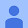Hugo Bracamontes Baltazar says:

Good!

2.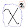Jay R says:

Good!

3.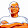BeeRich33 says:

Good!

4.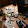Tanishq says:

Good!

5.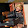Logan Flack says:

Please keep pumping out these videos! I like the pacing — explaining all the necessary basics so they seem intuitive, then launching into their more advanced applications. It may be a bit fast paced, but I just watch the video again if there is anything I missed the first time around. I’ll spread the word as much as I can.

1.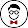Shawn Hymel says:

6.NEXTRONIC says:

good

7.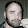Steven Cypherd says:

Very good, the Arduino is complicated. We need more training like this.

8.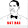Forest Trump says:

Hey when i try to upload a sketch to my redbot arduino i get this

avrdude: stk500_getsync() attempt 1 of 10: not in sync: resp=0x00
avrdude: stk500_recv(): programmer is not responding

like 10 times
What could be the problem, my drivers are updated i checked, am i using the wrong board? or programmer? Im using the Arduino/Genuino Uno board and the AVRISP mkll programmer

1.Shawn Hymel says:

Were you able to get this figured out? If you’re using an UNO, you should just need a USB cable. If you’re set on using the AVRISP mkii, it seems like you’ll need to do some extra work: https://forum.arduino.cc/index.php?topic=118089.0

2.Forest Trump says:

Shawn Hymel lol actually i just didnt turn the power on i didnt know the power switch was on the arduino ty for responding tho i appreciate it

3.Shawn Hymel says:

Forest Trump lol, glad it worked out. Sorry it took me so long to reply!

9.Krish Ath says:

Superb tutorial..thank you so much!@

1.Shawn Hymel says:

10.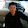iksan maulana says:

sir how i can to input the number of the variabel at serial monitor?

1.Shawn Hymel says:

Do you mean you want to read input from the Serial Monitor? If so, I recommend using the Serial.parseInt(), Serial.readString(), or Serial.readStringUntil() methods. See the Arduino reference guide for more information: https://www.arduino.cc/reference/en/language/functions/communication/serial/

2.iksan maulana says:

okay sir,but could i to write a number through serial monitor…

11.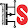Dwi Novianto says:

How to calculate type logaritmic operation in arduino.
I really need it.

12.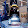adithmart says:

Im trying to divide…60 / .720 and I get the answer of 0….I can’t figure out how to get the result that I need in my code. I can do this .720 / 60 and I get a correct result, but the other equation does not work right.

13.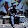_PurplePower_ mc playz says:

thanks a lot! this helped a ton.

14.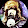S.S RADON says:

this helped me in automating coil wrapping as i need to keep the speed of one motor constant relative to the speed of the other motor in turns per second, which decrease as new layers are added because the perimeter of the spool increases.